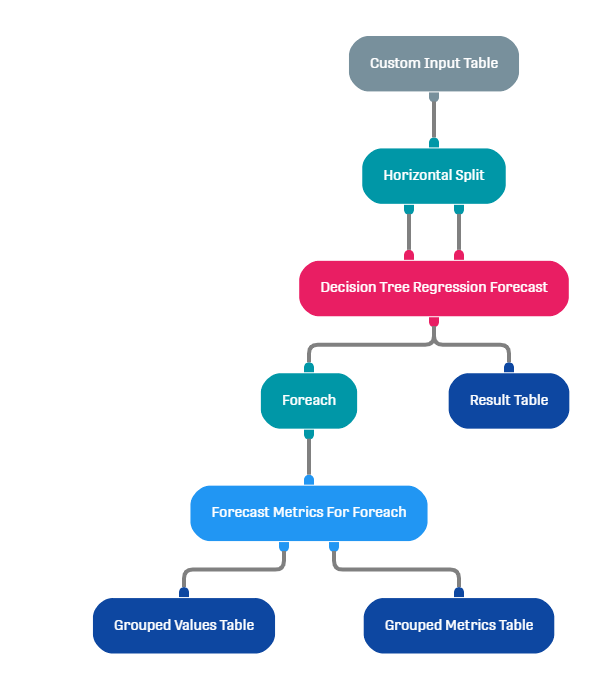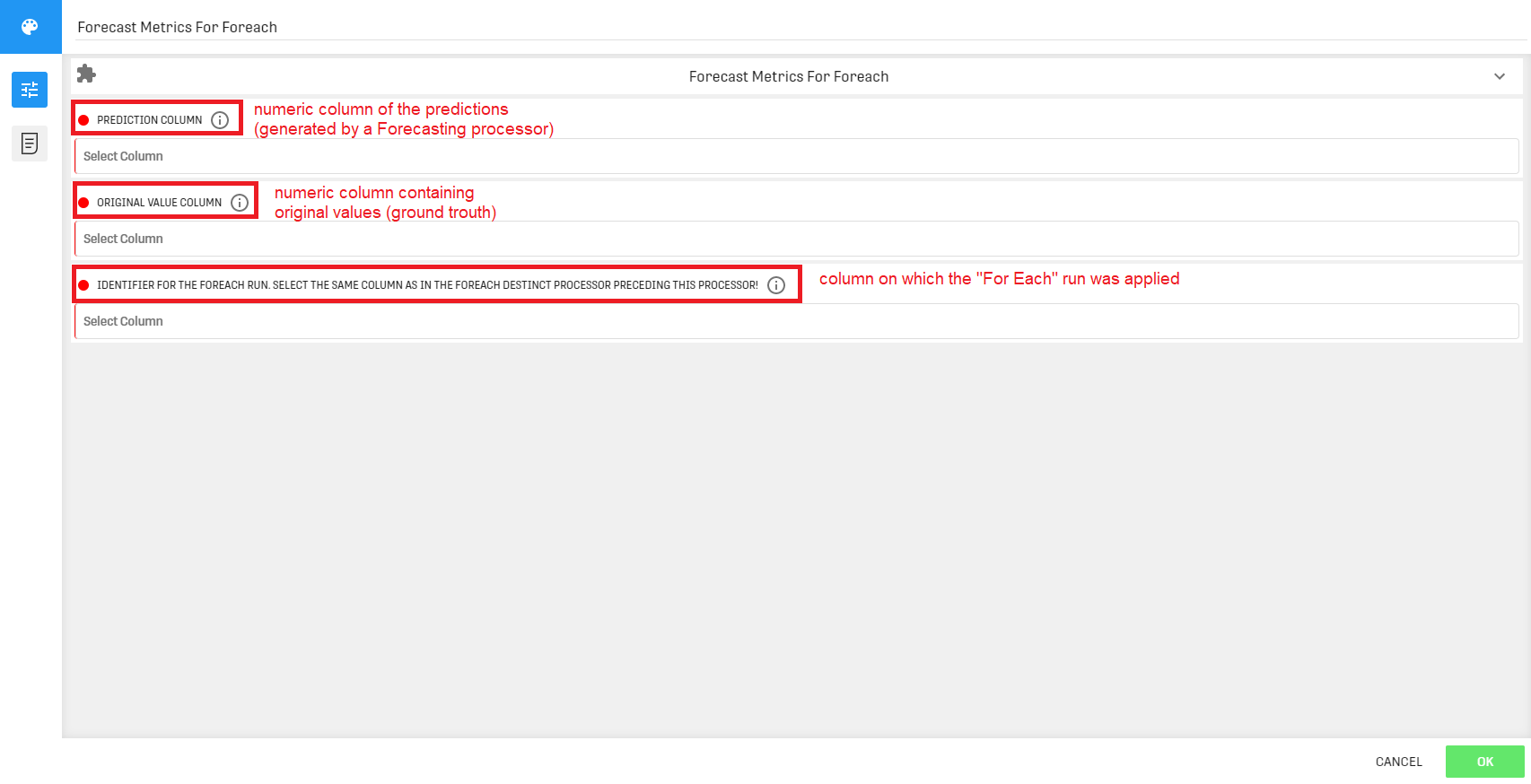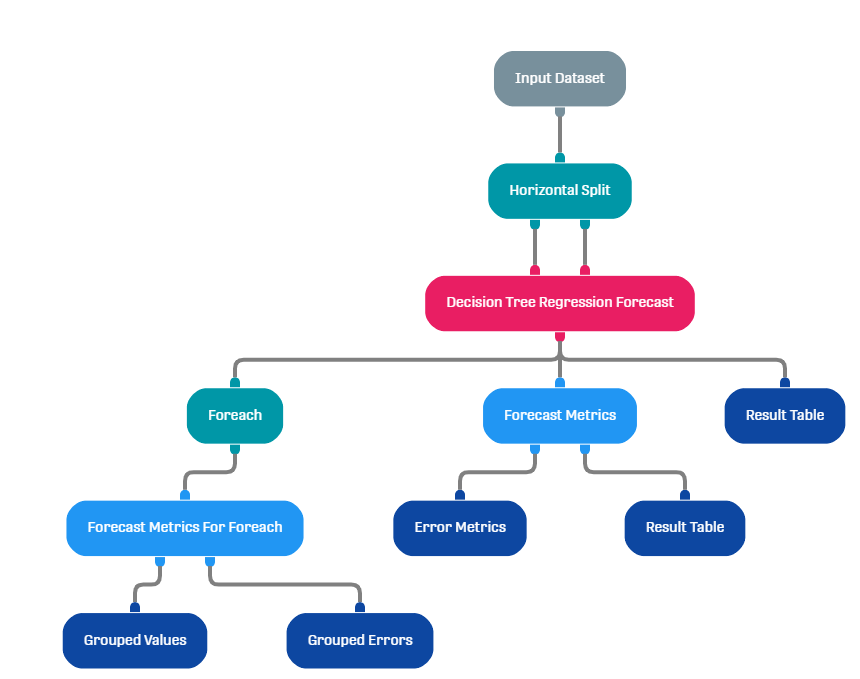# Forecast Metrics For Foreach Processor

Modified on Tue, 30 Nov 2021 at 11:44 AM

# Overview

This processor is used to calculate different error measures from forecasts generated in a Foreach branch (created by the Foreach Processor). It outputs a single-row dataset containing the forecast metrics foreach distinct value in the dataset.

NOTE THAT: It is very recommended to have a basic understanding of the functionality of "For Each" as well as the "Forecast Metrics" processors.

# Input

This processor requires a Dataset that has been processed by a "For Each" processor. Additionally this Dataset should contain a forecast column and an original value column.

# Configuration

This processor should be linked to the output node of a For Each Processor. The latter should be linked to the output node of a Forecasting processor (such as Decision Tree Regression Forecast).

An example of a Workflow pipeline looks as follows:When opening the configuration menu of this processor, the following interface shows up:NOTE THAT: The same column in the For Each and in the Forecast Metrics For Foreach (third configuration field) processors should be selected.

# Output

This processor provides two output nodes:

• Left Node: returns the input Dataset grouped with respect to the values of the selected column in the Foreach processor (a drop-down menu is shown in the result table)
• Right Node: return the calculated error metrics Mean Squared Error (MSE), Root Mean Squared Error (RMSE) and Mean Average Error (MAE) grouped by the values of the same column selected in the Foreach processor (there is also a drop-down menu in the result table)
The output of the right node can help track the forecasting errors with respect to specific values of the selected column which can help understand correlation between this column and the column on which forecasting is performed on.Furthermore this can help detect Outliers.

# Example

In the following Workflow we used Custom Input Table to create a toy Dataset, Horizontal Split Processor to divide it into training and test sets which both will be fed to a Decision Tree Regression Forecast which output will be linked to a Result Table (direct visualization of the forecasting processor output). The output is linked to a Forecast Metrics Processor and a Foreach Processor. We then insert the Forecast Metrics For Foreach Processor.

The workflow looks as follows: